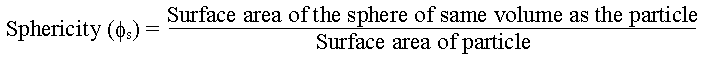### Sphericity of Cylinder

Calculate the sphericity of a cylinder of dia 1 cm and height 3 cm.

Calculations:Volume of particle = p rc2 h = p x 0.52 x 3 = 2.356 cm3

Radius of sphere of volume 2.356 cm3:

4 p rs3 / 3 = 2.356

rs = 0.8255 cm

Surface area of sphere of same volume as the particle = 4 p rs2 = 4 x p x 0.82552 = 8.563 cm2

Surface area of particle = 2 p rc (h + rc) = 2 x p x 0.5 x (3 + 0.5) = 10.996 cm2

Sphericity (f s) = 8.563/10.996 = 0.779

Sphericity could also be found from the formula,

Sphericity (f s) = 6 Vp / (DpSp)

Where Vp = volume of particle

Dp = Equivalent diameter of particle. (Equivalent diameter is defined as the diameter of a sphere of equal volume)

Sp = surface area of particle

Vp = p rc2 h = 2.356 cm3

Dp = 2 rs = 2 x 0.8255 = 1.651 cm

Sp = 2 p rc (h + rc) = 10.996 cm2

f s = 6 x 2.356 / (1.651 x 10.996) = 0.779

HOME